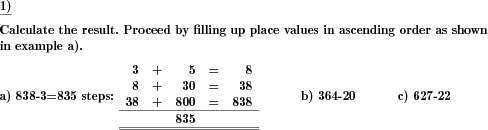Custom math worksheets at your fingertips# Details for problem "Subtraction by filling up place values"

Quickname: 4950

Elementary School, Primary School.

## Summary

Calculate difference by stepping up each place value.

## Example## Description

The difference of two positive whole numbers has to be calculated. In this approach this is achieved by determining the number of steps it takes to advance each of the subtrahend's place values up to the minuend's place value. This is done in ascending order, from right to left.

A sample problem with the answer shown can be given to illustrate the task.

For the minuend and subtrahend, the number of places can be selected. For the minuend's places, it can be specified that:

• any combination is permitted, as long as the subtrahend is smaller than the minuend.
• in all places, the minuend is greater than the subtrahend. This way, there will be no carries.
• the minuend is a multiple of a power of ten. This means each subtrahend's place will only have to be filled up to ten.

The number of problems can be selected.

Download free printable worksheets for this math problem here. The worksheet contains the problems only, the solution sheet includes the answers. Just click on the respective link.

•Worksheet 1Solution sheet with answers
•Worksheet 2Solution sheet with answers
•Worksheet 3Solution sheet with answers

If you can not see the solution sheets for download, they may be filtered out by an ad blocker that you may have installed. If this is the case, please allow ads for this page and reload the page. The solution sheets will then reappear.

• Do these sample worksheets do not really fit?
• Do you need more math worksheets, with a different level of difficulty?
• Would you like to combine different problems on a worksheet and adjust them to your needs?
• As a teacher, you can put together your own worksheets using the automatically generated math problems provided.
With a free initial credit, you can start creating your own math worksheets in a few minutes.

You can try it for free! Register here, to create custom worksheets now!

## Customization options for this problem

Parameter
Possible values
Number of problems
1, 2, 3, 4, 5, 6, 7, 8, 9, 10
Minuend places
1, 2, 3, 4, 5, 6
Minuend structure
any, every place greater/equal, multiples of powers of 10
Subtrahend places
1, 2, 3, 4, 5, 6
Sample problem
Yes, No